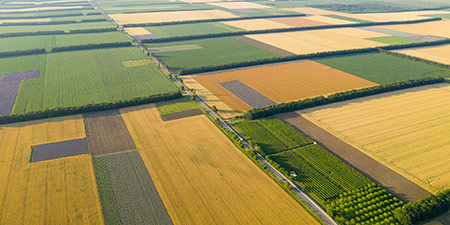# Square Foot (ft²) to Acre (ac)

Area Units ﹣ Converter Square Foot to Acre

Here you can convert the Area unit Square Foot into the unit Acre and vice versa you can convert Acre into Square Foot. By clicking the "Swap units" icon, you will always obtain the desired conversion in the calculation result, i.e., ft² to ac or ac to ft². With the following calculator you can also calculate any other Area unit.

A square foot (ft²; plural square feet) is equal to the area of a square with a side length of one foot (30.48 cm).

1 ft² = 1 ft × 1 ft

1 ft² = 30,48 cm × 30,48 cm

A foot is 12 inches long in the Anglo-American system and thus a square foot has 144 square inches. In the Anglo-American world, the size of land is indicated in land and property surveys using only the two area units, acre and square foot.

An acre (ac) is equal to one 160th part of a quarter section or one 640th part of a square mile. Thus, the acre has the area of a square with a side length of about 63.62 meters.

1 ac ≈ 63,62 m × 63,62 m

The unit acre (Old English æcer acre, field) originally referred to a strip of land that could be plowed in one day with a team of oxen.

The unit of area known as the acre is now a common unit for subdividing land areas, especially in America. These are initially divided into square miles (sections) and into quarters of these sections, namely quarters. A quarter section, in turn, consists of 160 acres. In the Anglo-American world, the size of properties in land and property surveys is indicated solely by the two area units acre and square foot.

1/640 square mile = 1/160 quarter = 1 acre = 4.840 square yards = 43.560 square feet = 6.272.640 square inches = 4.046,85642 m²

## Basis for conversion Square Foot (ft²) to Acre (ac) and vice versa

The abbreviation for the "Area unit Square Foot" is ft². The abbreviation for the "Area unit Acre" is ac.

## Formula for the conversion of Square Foot (ft²) to Acre (ac) and vice versa

The calculation from Square Foot to Acre shall be made using the following conversion formula:

Conversion formula Square Foot to Acre

Determine the number of Acre from Square Foot

Square Foot × 0.00024710538146717

## Formula for the conversion of Acre (ac) to Square Foot (ft²)

The calculation from Acre to Square Foot shall be made using the following conversion formula:

Conversion formula Acre to Square Foot

Determine the number of Square Foot from Acre

Acre × 4046.8564224

## Overview table : How many Square Foot are in a Acre ?

Square Foot ft² ⇒ Acre ac
0.04 ft²  are  0.00001 ac
0.05 ft²  are  0.00001 ac
0.06 ft²  are  0.00001 ac
0.07 ft²  are  0.00001 ac
0.08 ft²  are  0.00002 ac
0.09 ft²  are  0.00002 ac
0.10 ft²  are  0.00002 ac
0.20 ft²  are  0.00004 ac
0.30 ft²  are  0.00007 ac
0.40 ft²  are  0.00009 ac
0.50 ft²  are  0.00012 ac
0.60 ft²  are  0.00014 ac
0.70 ft²  are  0.00017 ac
0.80 ft²  are  0.00019 ac
0.90 ft²  are  0.00022 ac
1 ft²  corresponds to  0.00024 ac
2 ft²  are  0.00049 ac
3 ft²  are  0.00074 ac
4 ft²  are  0.00098 ac
5 ft²  are  0.00123 ac
6 ft²  are  0.00148 ac
7 ft²  are  0.00173 ac
8 ft²  are  0.00197 ac
9 ft²  are  0.00222 ac
10 ft²  are  0.00247 ac
20 ft²  are  0.00494 ac
30 ft²  are  0.00741 ac
40 ft²  are  0.00988 ac
50 ft²  are  0.01235 ac
60 ft²  are  0.01482 ac
70 ft²  are  0.01729 ac
80 ft²  are  0.01976 ac
90 ft²  are  0.02223 ac
100 ft²  are  0.02471 ac
200 ft²  are  0.04942 ac
300 ft²  are  0.07413 ac
400 ft²  are  0.09884 ac
500 ft²  are  0.12355 ac
600 ft²  are  0.14826 ac
700 ft²  are  0.17297 ac
800 ft²  are  0.19768 ac
900 ft²  are  0.22239 ac
1 000 ft²  are  0.24710 ac

## Overview table : How many Acre are in a Square Foot ?

Acre ac ⇒ Square Foot ft²
0.01 ac  are  40.46856 ft²
0.02 ac  are  80.93712 ft²
0.03 ac  are  121.40569 ft²
0.04 ac  are  161.87425 ft²
0.05 ac  are  202.34282 ft²
0.06 ac  are  242.81138 ft²
0.07 ac  are  283.27995 ft²
0.08 ac  are  323.74851 ft²
0.09 ac  are  364.21707 ft²
0.10 ac  are  404.68564 ft²
0.20 ac  are  809.37128 ft²
0.30 ac  are  1 214.05692 ft²
0.40 ac  are  1 618.74256 ft²
0.50 ac  are  2 023.42821 ft²
0.60 ac  are  2 428.11385 ft²
0.70 ac  are  2 832.79949 ft²
0.80 ac  are  3 237.48513 ft²
0.90 ac  are  3 642.17078 ft²
1 ac  corresponds to  4 046.85642 ft²
2 ac  are  8 093.71284 ft²
3 ac  are  12 140.56926 ft²
4 ac  are  16 187.42569 ft²
5 ac  are  20 234.28211 ft²
6 ac  are  24 281.13853 ft²
7 ac  are  28 327.99495 ft²
8 ac  are  32 374.85137 ft²
9 ac  are  36 421.70780 ft²
10 ac  are  40 468.56422 ft²
20 ac  are  80 937.12844 ft²
30 ac  are  121 405.69267 ft²
40 ac  are  161 874.25689 ft²
50 ac  are  202 342.82112 ft²
60 ac  are  242 811.38534 ft²
70 ac  are  283 279.94956 ft²
80 ac  are  323 748.51379 ft²
90 ac  are  364 217.07801 ft²
100 ac  are  404 685.64224 ft²
200 ac  are  809 371.28448 ft²
300 ac  are  1 214 056.92672 ft²
400 ac  are  1 618 742.56896 ft²
500 ac  are  2 023 428.21120 ft²
600 ac  are  2 428 113.85344 ft²
700 ac  are  2 832 799.49568 ft²
800 ac  are  3 237 485.13792 ft²
900 ac  are  3 642 170.78016 ft²
1 000 ac  are  4 046 856.42240 ft²

## Source information

As source for the information in the 'Area Units' category, we have used in particular:

## Last update

This page of the 'Area Units' category was last edited or reviewed by Michael Mühl on February 20, 2023. It corresponds to the current status.

### Changes in this category "Area Units"

• 14.11.2022: Publication of Area Converter together with associated texts.